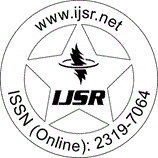International Journal of Science and Research (IJSR)
Call for Papers | Fully Refereed | Open Access | Double Blind Peer Reviewed

Research Paper | Mathematics | Iraq | Volume 7 Issue 1, January 2018

# Z-Nearly Prime Submodules

Abstract: Let R be a commutative ring with identity and N be a proper submodule N of R-moduLe M is called prime if whenever rxN, rR, xM, implies either xN or r [NM]. In this paper we say that N is Z-nearly prime submodule of R-moduLe M, if whenever fM^*=Hom (M, R), x M such that f (x) x N, then either x N+J (M) or f (x) [N+J (M) M], J (M) is the Jacobson radical of M. We prove some result of this type of submodules

Keywords: prime submodule, Z-prime submodule, nearly prime submodule, nearly regular module

Edition: Volume 7 Issue 1, January 2018,

Pages: 796 - 799